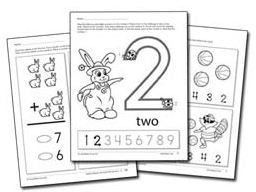# Teaching Subtraction in Special Ed With Touch Math Numerals

## Teaching the Number Line

Before you teach students how to subtract using Touch Math numerals, you will teach them the Touch Math number line. This means showing and teaching students the touch points for each numeral one through nine. The Touch Math website has free resources that explain to you and your students with special needs the touch points.

You may need to spend a few weeks with students just learning these touch points before you work on subtraction skills. Instead of students with special needs spending class time or homework time memorizing subtraction facts, they can work on these touch points while other students are memorizing.

## Teaching SubtractionWhen teaching subtraction with Touch Math, you are actually teaching students to count backward from the larger number. For example if the problem is 15 minus 7, students will start at 15 and count backward 7, using the Touch Math numeral touch points, until they reach the answer of 8.

With the Touch Math number line, seven has certain touch points. When the student touches the first touch point, he would say “14.” When he touches the second touch point, he would say, “13,” and so on. Because students are counting backward to work a subtraction problem, they need to practice counting backward from 20 until it becomes easy for them; sometimes, they will get the wrong answer just because they made a mistake counting backward. If students know the Touch Math number line well, this will also help them to focus on counting backward while working subtraction problems.

## Free Resources

As mentioned, the Touch Math website has several free resources you can download and use to see if this program will work for your students with special needs or tactile learners who are having difficulties with math facts. The website has free materials organized by grade level, and these can also show you the developmental stages of Touch Math. For example, the Kindergarten free subtraction worksheets, ask students to count backward from 8 to 4.

Counting backward is a main skill of Touch Math subtraction, and so this worksheet helps students work on that skill. There are also sample scripted directions for teachers.The other two worksheets focus on one type of subtraction problem and how to use the Touch Math numerals to solve it. Again, scripted directions are provided for special education teachers.

The first grade and second grade free subtraction worksheets build on the skills that students learn when they are first beginning the Touch Math program. The students in these grades use Touch Math numerals to subtract two-digit and three-digit numbers and to work on place value. So, if you have students who are struggling with harder subtraction problems, then you can also use the Touch Math program with them. This includes subtraction problems with borrowing or regrouping. The counting backward process is basically the same no matter what the level of the subtraction problem.

The Touch Math website has free sample materials up to the upper elementary grades, and you can use these materials and the Touch Math numerals to help older students with subtraction problems.